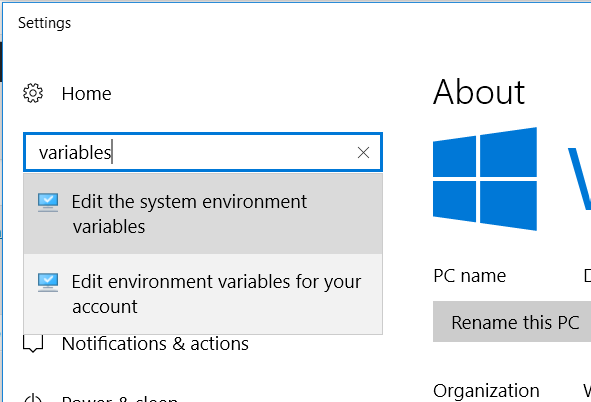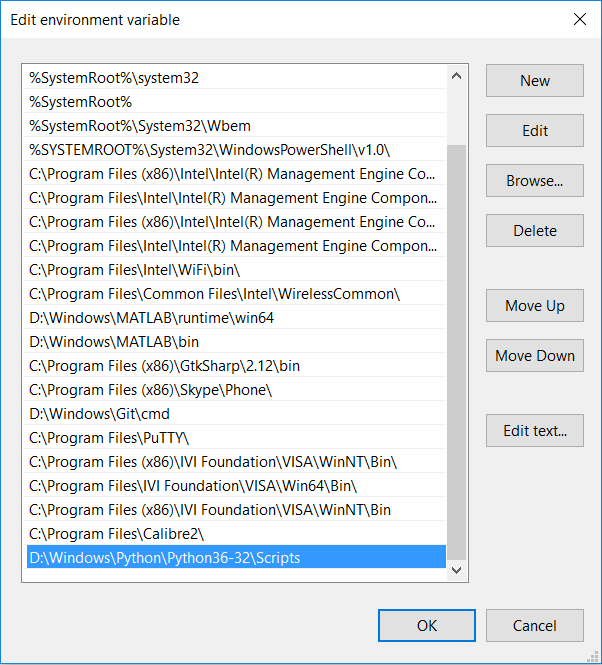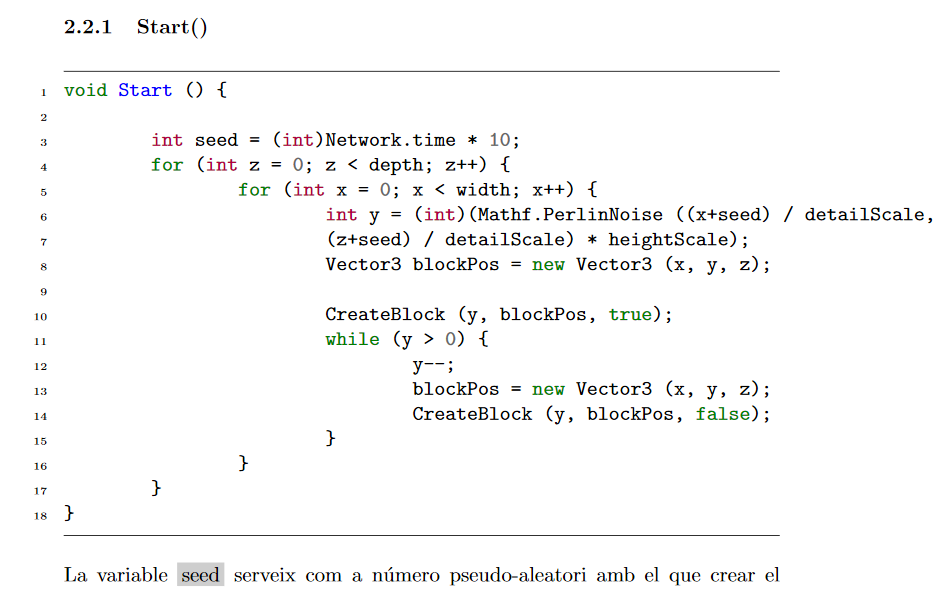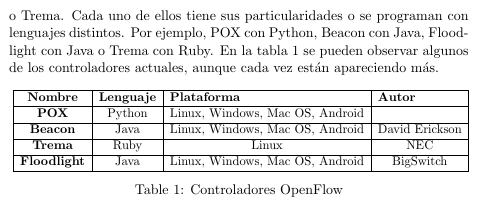Categorías

## Permanent record

The freedom of a country can only be measured by its respect for the rights of its citizens, and it’s my conviction that these rights are in fact limitations of state power that define exactly where and when a government may not infringe into that domain of personal or individual freedoms that during the American Revolution was called «liberty» and during the Internet Revolution is called «privacy».

Edward Snowden, Permanent Record (2019)

Categorías

## Inner product in vector space

The inner product is an operation that measures the similarity between vectors.  In a general way, the inner product could be defined as an operation of 2 operands, which are elements of a vector space. The result is a scalar in the set of the complex numbers:

$\left \langle \cdot, \cdot \right \rangle : V \times V \rightarrow \mathbb{C}$

## Formal properties

For $$x, y, z \in V$$ and $$\alpha \in \mathbb{C}$$, the inner product must fulfill the following rules:

To be distributive to vector addition:

$$\left \langle x+y, z \right \rangle = \left \langle x, z \right \rangle + \left \langle y, z \right \rangle$$

Conmutative with conjugate (applies when vectors are complex):

$$\left \langle x,y \right \rangle = \left \langle y, x \right \rangle^*$$

Distributive respect scalar multiplication:

$$\left \langle \alpha x, y \right \rangle = \alpha^* \left \langle x, u \right \rangle$$

$$\left \langle x, \alpha y \right \rangle = \alpha \left \langle x, u \right \rangle$$

The self inner product must be necessarily a real number:

$$\left \langle x, x \right \rangle \geq 0$$

The self inner product can be zero only when the element is the null element:

$$\left \langle x,x \right \rangle = 0 \Leftrightarrow x = 0$$

## Inner product in $$\mathbb{R}^2$$

The inner product in $$\mathbb{R}^2$$ is defined as follows:

$$\left \langle x, y \right \rangle = x_0 y_0 + x_1 y_1$$

In self inner product represents the squared norm of the vector:

$$\left \langle x, x \right \rangle = x^2_0 + y^2_0 = \left \| x \right \|^2$$

## Inner product in finite length signals

In this case, the inner product is defined as:

$\left \langle x ,y \right \rangle = \sum_{n= 0}^{N-1} x^*[n] y[n]$

Categorías

## Properties of vector spaces

Vector spaces must meet the following rules:
$$x + y = y + x$$

$$(x+y)+z = x + (y + z)$$

Scalar multiplication to be distributive with respect to vector addition:
$$\alpha\left(x + y \right) = \alpha x + \alpha y$$

Scalar multiplication to be distributive with respect to vector the addition of field scalars:
$$\left( \alpha + \beta \right) x = \alpha x + \beta y$$

Scalar multiplication to be associative:
$$\alpha\left(\beta x \right) = \left(\alpha \beta \right) x$$

It must exist a null element:
$$\exists 0 \in V \ \ | \ \ x + 0 = 0 + x = x$$

It must exist an inverse element for every element in the vector space:
$$\forall x \in V \exists (-x)\ \ | \ \ x + (-x) = 0$$

Categorías

## Instalar minted en Windows 10

minted es un paquete de LaTeX que permite añadir código a tu documento y mostrarlo resaltado. Minted utiliza Python para compilar, por lo que el primer paso es instalar Python 2.6 o superior.

1. Instalar Python
2. Añadir la ruta de Python a las variables del entorno de Windows.
1. Botón derecho en el icono de Windows.
2. Sistema
3. En el buscador añadir «variables» y clickar en «Editar variables del sistema».4. Clickar en Variables del entorno.
5. En Variables del sistema, añadir a la entrada Path la localización de la carpeta Scripts de Python. En mi caso está en D:\Windows\Python\Python36-32\Scripts. Es muy probable que este paso no sea necesario en tu sistema y que al instalar Python ya se haya añadido la ruta a las variables del sistema. Si al ejecutar python en CMD da un error, entonces este paso es obligatorio.6. Instalar pip.
7. Instalar pygments:
1. Abrir CMD en Windows y escribir: pip install Pygments
2. Probar que se ha instalado correctamente escribiendo en CMD pygmentize -h
8. Ahora desde TexStudio hay que modificar la llamada al compilador de LaTeX.
1. Opciones > Configurar TeXStudio > Órdenes
2. Modificar las entradas LaTeX y PdfLaTeX por: latex.exe -src -interaction=nonstopmode -shell-escape %.tex y pdflatex.exe -synctex=1 -interaction=nonstopmode -shell-escape %.tex respectivamente. De esta manera se añade la etiqueta -shell-escape después de -interaction=nonstopmode
3. Cerrar TeXStudio
4. Abrir de nuevo

Y si no ha habido ningún problema en los pasos anteriores, se debería poder compilar utilizando minted. Para más información, visitad la guía de instalación de minted.\begin{minted}[mathescape,
linenos,
numbersep=8pt,
gobble=0,
frame=lines,
framesep=2mm]{csharp}
public static int width = 64;	// Landscape width
public static int height = 128;	// Landscape height
public static int depth = 64;	// Landscape depth
public int heightScale = 20;	// Maximum terrain's height
public float detailScale = 25.0f;
public Transform vrCamera;
public GameObject grassBlock;	// Grass block object
Block[,,] worldBlocks = new Block[width, height, depth];// 3D array with blocks
\end{minted}

Categorías

## Encontrar posición de un número dentro de una matriz en MATLAB

maxdB = max(meanFrequency);
maxPos = find(meanFrequency == maxdB);
Categorías

## Desajuste en la referencia a figuras, ecuaciones o tablas en LatexSi el número que aparece como referencia al referenciar un objeto (imagen, ecuación, tabla, etc.) mediante el comando \ref{} no coincide con el del elemento, es porque la sentencia \caption{} aparece después de la de \label{}.

\caption{Controladores OpenFlow}
\label{table:controllers}


Esto ocurre porque elementos numerados como /section, \begin{equation} o \caption utilizan un contador para saber qué número es el siguiente elemento. Este contador es \refstepcounter y sirve para incrementar el contador que lleva la cuenta del número de índice de un capítulo, número de ecuación o número de figura. Cuando se escribe una etiqueta \label se guarda esa información en el archivo .aux con el fin de que cuando se escriba \ref en el documento, sepa cuál de todos los contadores debe mostrar para esa referencia. Cuando se está dentro de un entorno como por ejemplo \begin{equation} o \begin{figure} se le está diciendo a \label que incremente el contador de ese entorno. Lo mismo ocurre con table. Por tanto, cuando se pone \label antes que \caption, se incrementa el contador de \label y se muestra el número erróneo en \ref

Para ver los cambios puede que sea necesario recompilar un par de veces.Categorías

## Progresiones

Progresión geométrica:

$\sum_{k=N_1}^{N_2} a r^k = a \frac{r^{N_1}-r^{N_2+1}}{1-r}$

$\sum_{k=0}^{\infty} a r^k = \frac{a}{1-r}\text{ para |r|<1}$

Progresión aritmética de $$n$$ términos:

$\sum_{k=1}^{M} k = \frac{n \left(k_1 + k_M\right)}{2}$

Categorías

Coseno de la suma:

$\cos{(a+b)} = \cos{a} \cos{b} – \sin{a}\sin{b}$

$\cos{(a-b)} = \cos{a} \cos{b} + \sin{a}\sin{b}$

Seno de la suma:

$\sin{(a+b)} = \sin{a}\cos{b} + \sin{b}\cos{a}$

$\sin{(a-b)} = \sin{a}\cos{b} – \sin{b}\cos{a}$

Coseno del ángulo doble:

$\cos{2a} = \cos^2{a} – \sin^2{a}$

Seno del ángulo doble:

$\sin{2a} = 2\sin{a}\cos{a}$

Diferencia de senos:

$\sin{a} – \sin{b} = 2\cos{\frac{a+b}{2}}\cos{\frac{a-b}{2}}$

Categorías

## Forzar a una imagen aparecer en la sección correspondiente en LaTeX

En LaTeX hay veces que al compilar el documento la imagen aparece en la página siguiente o incluso antes. Para evitar esta situación basta con utilizar unos modificadores en la definición de la figura que son [!h]

• !: fuerza a sobreescribir cualquier configuración anterior ya descrita.
• h: fuerza a aparecer en esa misma sección la imagen. h viene de here

Ejemplo:

\begin{figure}[!h]
\caption{Impedancia de entrada desde 285 MHz hasta 310 GHz para ambos resonadores representada en la carta de Smith}
\label{fig_zin_smith}
\end{figure}
Categorías

## Coeficiente binomial

$$\binom{n}{i}$$ es el número de subconjuntos de $$i$$ elementos que se puede hacer con un conjunto $$n$$ elementos.

$$n$$: número total de elementos
$$i$$: longitud de los subconjuntos
$\binom{n}{i} = \frac{n!}{i! \left( n-k \right)! }$
Esto nos sirve también para poder entender el ensayo de Bernoulli. Este nos dice que si el resultado de un experimento ocurre con probabilidad $$k$$ veces es:
$P_n \left( k \right) = \binom{n}{k} P_A^k \left( 1-P_A \right)^{n-k}$